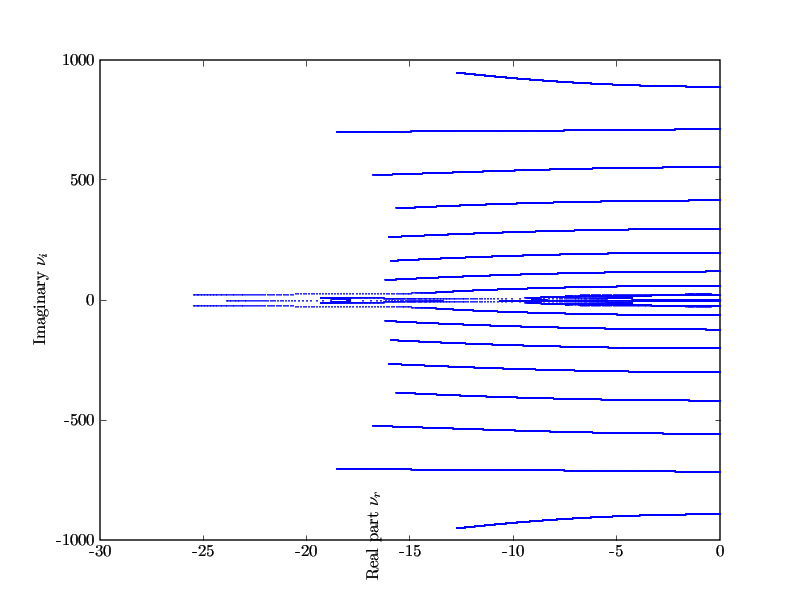# Problems with xlabel

Hi all,

I have some problems with xlabel. Sometimes the text is rotated (see
ex3.png), but for what reason ?

figure(3)
plot(real(data_eig),imag(data_eig),'b.')
xlabel(r'Real part $\nu_r$')
ylabel(r'Imaginary $\nu_i$')
savefig('ex3.png')

NilsI can't reproduce this behavior, but I have found that TeX strings are sometimes not rendered correctly unless I specify a fontsize parameter: xlabel(r'Real part $\nu_r$', fontsize=16) for example.

-gary

Nils Wagner wrote:

···

Hi all,

I have some problems with xlabel. Sometimes the text is rotated (see
ex3.png), but for what reason ?

figure(3)
plot(real(data_eig),imag(data_eig),'b.')
xlabel(r'Real part $\nu_r$')
ylabel(r'Imaginary $\nu_i$')
savefig('ex3.png')

Nils

------------------------------------------------------------------------

a fontsize parameter: xlabel(r'Real part $\nu_r$', > fontsize=16)